# Brain proteome in Alzheimer's and Parkinson's disease¶

By Tim Woelfle, 27/08/2019

This is an exploratory analysis of the openly available brain proteome dataset by Ping et al., described in their 2018 article: Ping, L., Duong, D.M., Yin, L., Gearing, M., et al. (2018) Global quantitative analysis of the human brain proteome in Alzheimer’s and Parkinson’s Disease. Scientific Data. [Online] 5, 180036. Available from: doi:10.1038/sdata.2018.36.

It contains mass-spectrometry based proteomes from 40 brain tissue donors: 10 controls, 10 Alzheimer's patients (AD), 10 Parkinson's patients (PD) and 10 patients with both (ADPD). Samples were analyzed from the frontal cortex (Brodmann area 9) and the cingulate gyrus (Brodmann area 24). This exploratory analysis focuses on Alzheimer's Disease and the cingulate gyrus but could be repeated in the same way for Parkinson's Disease and / or the frontal cortex. An overview of most methods used here is given in this review-paper on statistical OMICS analysis (subscription only).

In :
import numpy as np
import pandas as pd
import seaborn as sns

import umap

import statsmodels.formula.api as smf
import statsmodels.stats.multitest as smm

from sklearn.decomposition import PCA
from sklearn.cross_decomposition import PLSRegression
from sklearn.linear_model import LogisticRegressionCV
from sklearn.model_selection import train_test_split
from sklearn import metrics


## 1. Sample overview¶

samples_trait_cingulate.csv is derived from the second sheet "anterior cingulate gyrus" of samples trait.xlsx

In :
samples = pd.read_csv("samples_trait_cingulate.csv")

Out:
Case number Primary neuropathologic diagnosis Overall CERAD Braak PMI (hr) Age of Onset Age at Death Duration (yr)2 ApoE Status Race Sex
0 OS98-11 AD 1 3 6 6.0 56.0 65 9.0 E4/4 w f
1 OS00-11 AD 2 3 5 4.0 49.0 55 6.0 E3/3 w m
2 OS00-32 AD 3 3 6 3.5 55.0 62 7.0 E3/4 w m
3 OS03-163 AD 4 3 6 4.5 51.0 55 4.0 E3/4 w f
4 E04-186 AD 5 3 6 7.0 59.0 72 13.0 E3/4 w f

### 1.1 Sex distribution by condition¶

In :
samples["Condition"] = pd.Series(["AD","AD","Control","Control","PD","PD","ADPD","ADPD"]*5)
pd.crosstab(samples.Condition, samples.Sex, margins=True).plot.bar()

Out:
<matplotlib.axes._subplots.AxesSubplot at 0x7f36cd4937f0>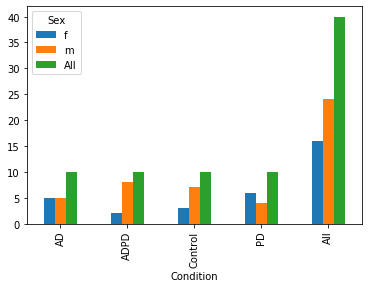### 1.2 Age at death by condition¶

In :
samples.boxplot("Age at Death", by="Condition")

Out:
<matplotlib.axes._subplots.AxesSubplot at 0x7f36cd493828>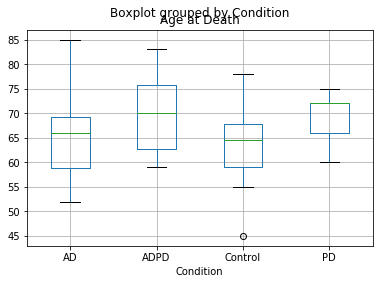## 2. Cingulate proteome preprocessing¶

cingulate.csv is derived from the second sheet "anterior cingulate gyrus" of TMT_Summary_Data.xlsx with the first two rows removed

In :
proteome = pd.read_csv("cingulate_.csv").set_index("Accession")
proteome_dict = proteome.loc[:,"Gene":"Description"]

Out:
Accession Q5VT66-2 D6RGC4 Q969Z3 Q9NX47 Q9H992 A6NNE9 Q15019-2 C9IY94 Q9UH03-2 Q9UH03 ... Q8TBC5-3 Q96LW9 A7E2V4-2 S4R3B3 O43264 Q9C0D3 Q15942 O43149 O43149-3 Q8IYH5
AD1 1.124580 0.666667 0.948162 1.067472 NaN NaN 1.076074 1.148094 0.911617 0.0 ... 1.454445 0.877273 1.310105 1.524927 0.942656 1.108474 0.844916 1.002873 1.250597 0.0
AD2 0.881134 0.651515 0.713516 0.932528 NaN NaN 1.344181 1.336144 0.837435 0.0 ... 0.967641 0.759091 1.017422 0.885630 0.930584 0.966762 0.951352 1.005534 1.183771 0.0
CTL1 0.795806 0.621212 0.675168 0.946626 NaN NaN 1.027415 1.054985 0.736138 0.0 ... 1.012096 0.790909 1.486643 3.300098 0.980885 0.831706 0.622753 1.029573 1.031026 0.0
CTL2 0.960637 1.181818 1.090438 0.958711 NaN NaN 0.874709 0.892595 1.033973 0.0 ... 1.170908 1.268182 1.094077 0.838710 1.018360 0.990585 1.248980 1.085847 1.183771 0.0
PD1 1.125191 1.484848 1.186200 1.105740 NaN NaN 0.854764 0.801320 0.965995 0.0 ... 0.556865 5.927273 1.279907 1.090909 1.072435 1.013882 1.336909 0.998395 1.011933 0.0

5 rows × 10695 columns

### 2.1 Investigate missing values¶

A single value of 0 means the protein was identified but not successfully quantified, if the whole batch is 0 it means that one of the GIS (global internal standards) was missing and the measurement is therefore considered unreliable, see sheet "Key" in TMT_Summary_Data.xlsx. There are 5 batches of 8 subjects each. In either case we consider them NA.

In :
(proteome.isna() | (proteome == 0)).sum().value_counts().plot.bar()

Out:
<matplotlib.axes._subplots.AxesSubplot at 0x7f36cd9dcfd0>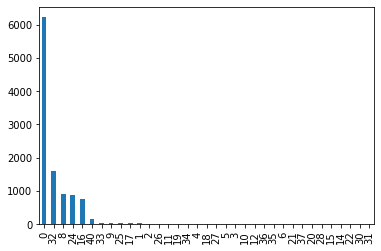The barplot shows that most proteins with NA values are missing for at least one whole batch (8/16/24/32/40 subjects), so imputation is risky. We try a complete case analysis first, with nearly 60% of all proteins.

In :
(proteome>0).all().sum() / proteome.shape

Out:
0.5825151940158952

### 2.2 Remove proteins with NAs and normalize¶

Normalization to mean 0 and standard deviation 1 per column is important for PCA.

In :
proteome = proteome.loc[:, (proteome>0).all()]
proteome = (proteome - proteome.mean()) / proteome.std()

Out:
Accession Q5VT66-2 Q969Z3 Q15019-2 C9IY94 Q9UH03-2 Q99719 G3XAH0 Q14141 Q16181-2 Q16181 ... Q14966 Q9Y5V0 Q8NHG8 O75312 Q8TBC5-3 A7E2V4-2 O43264 Q9C0D3 Q15942 O43149
AD1 1.006041 -0.330660 0.585419 0.814268 -0.931852 -0.891949 -1.172335 -0.301688 0.123567 -1.414264 ... 0.498701 -1.136658 -0.355748 -1.654166 2.035214 1.657337 -0.670185 1.475818 -0.876639 0.128153
AD2 -0.975074 -1.885644 2.600928 1.950324 -1.731064 -0.763846 -1.704209 -1.885033 0.411533 -0.762315 ... 0.934354 0.907615 -0.608691 -1.273378 -0.147662 0.146033 -0.872731 -0.271251 -0.308122 0.157057
CTL1 -1.669458 -2.139778 0.219625 0.251776 -2.822402 -1.984050 -2.551838 -2.368348 -2.718866 -1.922959 ... -1.468940 -0.865875 0.016641 1.134082 0.051678 2.568918 -0.028791 -1.936258 -2.063302 0.418108
CTL2 -0.328097 0.612196 -0.928350 -0.729263 0.386378 0.742056 0.862101 1.205374 0.812045 0.573740 ... -0.371849 -1.600656 -0.608691 -1.474000 0.763807 0.541851 0.599944 0.022447 1.281627 1.029217
PD1 1.011008 1.246803 -1.078290 -1.280682 -0.345995 0.056382 0.034887 0.910110 -0.906297 0.063583 ... 2.633783 -0.188916 -0.109831 -1.117601 -1.989617 1.501409 1.507179 0.309666 1.751289 0.079521

5 rows × 6230 columns

Distributions look more or less normal, don't seem to need log-transformation.

In :
proteome.iloc[:,0:16].hist()

Out:
array([[<matplotlib.axes._subplots.AxesSubplot object at 0x7f36cd9dc710>,
<matplotlib.axes._subplots.AxesSubplot object at 0x7f36cdd6e0f0>,
<matplotlib.axes._subplots.AxesSubplot object at 0x7f36cde29eb8>,
<matplotlib.axes._subplots.AxesSubplot object at 0x7f36cdf459b0>],
[<matplotlib.axes._subplots.AxesSubplot object at 0x7f36cddfa1d0>,
<matplotlib.axes._subplots.AxesSubplot object at 0x7f36cde0f5c0>,
<matplotlib.axes._subplots.AxesSubplot object at 0x7f36cdecf668>,
<matplotlib.axes._subplots.AxesSubplot object at 0x7f36cdd3bcc0>],
[<matplotlib.axes._subplots.AxesSubplot object at 0x7f36cdd3b0f0>,
<matplotlib.axes._subplots.AxesSubplot object at 0x7f36cc2e5278>,
<matplotlib.axes._subplots.AxesSubplot object at 0x7f36cd9293c8>,
[<matplotlib.axes._subplots.AxesSubplot object at 0x7f36cccfc668>,
<matplotlib.axes._subplots.AxesSubplot object at 0x7f36ccb5c7b8>,
<matplotlib.axes._subplots.AxesSubplot object at 0x7f36cdab2908>,
<matplotlib.axes._subplots.AxesSubplot object at 0x7f36cd9b3a58>]],
dtype=object)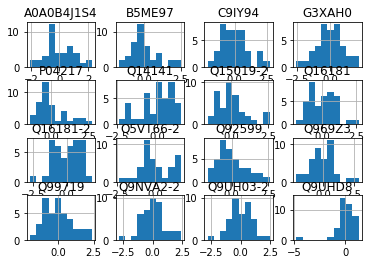## 3. Dimensionality reduction¶

### 3.1 Principal component analysis¶

The first PCA component of the cingulate proteome explains 22.3% of the variance and the second component 13.4%.

In :
pca_model = PCA()
pca = pca_model.fit_transform(proteome)[:,0:2]
print(pca_model.explained_variance_ratio_[0:2])
pd.Series(np.cumsum(pca_model.explained_variance_ratio_)).plot()

[0.18717674 0.10779146]

Out:
<matplotlib.axes._subplots.AxesSubplot at 0x7f36cdeb9128>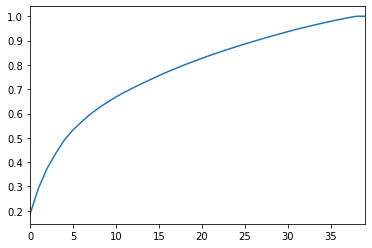In the PCA plot we can already see that AD+ADPD cluster more to the right whereas Control+PD cluster more to the left. However, these two components only capture roughly 30% of the variance in the data, let's see if UMAP produces a different result.

In :
# can't color categorically with pandas alone, so use seaborn
#pd.DataFrame(pca, columns=["PC1", "PC2"]).plot.scatter(x="PC1", y="PC2")
sns.scatterplot("PC1", "PC2", data=pd.DataFrame(pca, columns=["PC1", "PC2"]),
hue=samples.Condition)

Out:
<matplotlib.axes._subplots.AxesSubplot at 0x7f36cdb97c50>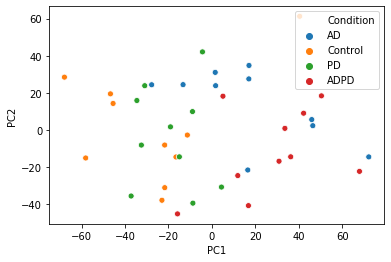### 3.2. UMAP¶

UMAP is a modern dimensionality reduction technique similar to t-SNE, able to shrink all of the data to two components. See more details here: https://github.com/lmcinnes/umap

Again, we can already appreciate that AD+ADPD seem to cluster together (top-right) whereas Control+PD seem to be clustered more in the bottom-left.

In :
embedding = umap.UMAP().fit_transform(proteome)
sns.scatterplot("UMAP 1", "UMAP 2", data=pd.DataFrame(embedding, columns=["UMAP 1", "UMAP 2"]),
hue=samples.Condition)

Out:
<matplotlib.axes._subplots.AxesSubplot at 0x7f36ba504da0>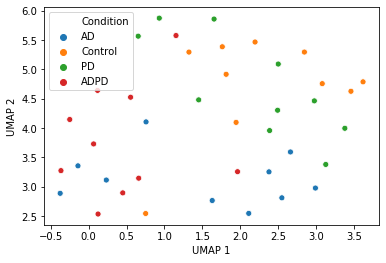### 3.3. Partial least squares¶

PLS can be considered both a dimensionality reduction method and a supervised learning algorithm. Whereas PCA & UMAP are agnostic of the outcome variable, PLS is fitting components to maximize variance not just in the input space X (proteome) but also in the outcome space Y, which is a 40x4 binary (dummy) matrix representing Controls, AD, PD & ADPD.

So this plot shows fairly good separability of the four classes when trained on the outcome data but we see that Alzheimer's (AD/ADPD) separates better from controls than does Parkinson's only (PD).

In :
pls = PLSRegression(n_components=2).fit(proteome, pd.get_dummies(samples.Condition))
sns.scatterplot("PLS 1", "PLS 2", data=pd.DataFrame(pls.x_scores_, columns=["PLS 1", "PLS 2"]),
hue=samples.Condition)

Out:
<matplotlib.axes._subplots.AxesSubplot at 0x7f36bab439e8>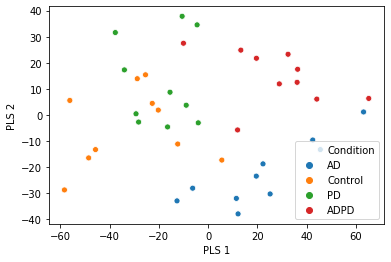## 4. Univariate analysis¶

This can be considered a "PWAS", a Proteome Wide Association Study". There are two ways of modelling this: Linear Regression and Logistic Regression

### 4.1 Multiple univariate linear regression¶

Every protein-quantity is tested as dependent variable against the two binary phenotypes Alzheimer's (including AD and ADPD patients) and Parkinson's (including PD and ADPD patients) in two separate univariate models. Results are saved to AD_univariate_linreg.csv and PD_univariate_linreg.csv

In :
AD = np.array([1,1,0,0,0,0,1,1]*5) # AD, ADPD: True; Control, PD: False

PDuniv = proteome_dict.loc[proteome.columns,:"Gene"]
for protein in proteome.columns:
lr = smf.ols("Q('" + protein + "') ~ AD", data=proteome).fit()
lr = smf.ols("Q('" + protein + "') ~ PD", data=proteome).fit()
PDuniv.loc[protein, "coef"] = lr.params
PDuniv.loc[protein, "pval"] = lr.pvalues

# Sort outcome by p-value
PDuniv = PDuniv.sort_values("pval")

PDuniv.to_csv("PD_univariate_linreg.csv")

In :
ADuniv.head(20)

Out:
Gene coef pval
Accession
Q9H4F8-2 SMOC1 1.802782 2.298555e-16
E9PLM6 MDK 1.663440 9.533849e-12
P05067 APP 1.625795 6.988686e-11
A0A087WWT2 NRN1 -1.583374 5.054728e-10
Q99784 OLFM1 -1.546295 2.363332e-09
Q8NAA5 LRRC75A -1.540159 3.006388e-09
Q96NI6 LRFN5 -1.529536 4.519406e-09
Q14571 ITPR2 1.509881 9.337636e-09
Q96CG8 CTHRC1 1.506727 1.045667e-08
Q13490 BIRC2 -1.504626 1.126973e-08
O75116 ROCK2 -1.498640 1.392172e-08
A6NHX0 GATSL2 -1.495699 1.542738e-08
P41240 CSK 1.495476 1.554758e-08
P01111 NRAS -1.492218 1.740487e-08
P31431 SDC4 1.490926 1.819681e-08
Q15223 PVRL1 -1.480379 2.603719e-08
H3BS89 TMEM178B -1.462102 4.745540e-08
F2Z2J1 RPS6KA2 1.459791 5.110624e-08
Q93009 USP7 -1.457857 5.436078e-08
Q15435 PPP1R7 -1.457113 5.566268e-08

#### 4.1.1 Significance tests and volcano plot¶

132 proteins are significant after Bonferroni correction, and an additional 1491 after FDR correction. The volcano plot looks U-shaped because the input matrix is normalized to mean 0 and std 1. See 2_compare_univariate_results.ipynb and 2_compare_univariate_results.html for interactive volcano plots.

In :
ADuniv["Significant"] = "False"

Out:
False         4607
FDR           1491
Bonferroni     132
Name: Significant, dtype: int64
In :
sns.scatterplot(ADuniv.coef, -np.log10(ADuniv.pval), hue=ADuniv["Significant"], edgecolor="none", alpha=0.3)

Out:
<matplotlib.axes._subplots.AxesSubplot at 0x7f36bacf6278>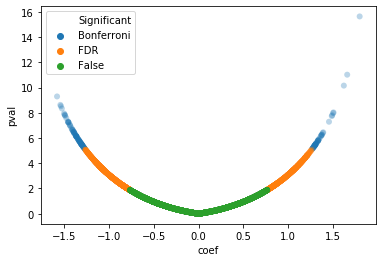#### 4.1.2 Biological interpretation¶

Among the Bonferroni significant top hits are two of Alzheimer's Disease hallmark proteins:

Another hallmark protein is not significant, probably because only its NAC fragment is associated with Alzheimer's:

The most significant protein however, encoded by the SMOC1 gene, has only recently been associated with Alzheimer's in a proteomic analysis of cerebrospinal fluid:

• SPARC-related modular calcium-binding protein 1, encoded by SMOC1 gene, UniProt ID Q9H4F8-2
In :
ADuniv.loc[("Q9H4F8-2", "P05067", "P10636", "P37840"),:]

Out:
Gene coef pval Significant
Accession
Q9H4F8-2 SMOC1 1.802782 2.298555e-16 Bonferroni
P05067 APP 1.625795 6.988686e-11 Bonferroni
P10636 MAPT 1.379071 5.446650e-07 Bonferroni
P37840 SNCA -0.408184 2.006676e-01 False

### 4.2 Multiple univariate logistic regression¶

For performing logistic regression, we need a binary dependent variable. Thus, this time we use AD / PD status as dependent (outcome) variable and protein-quantity as independent (input) variable in two separate univariate models per protein.

In :
ADuniv_log = proteome_dict.loc[proteome.columns,:"Gene"]
PDuniv_log = proteome_dict.loc[proteome.columns,:"Gene"]

for protein in proteome.columns:
#protein = proteome.columns
PD_logit = smf.logit("PD ~ Q('" + protein + "')", data=proteome).fit(disp=False, method='lbfgs')
PDuniv_log.loc[protein, "coef"] = PD_logit.params
PDuniv_log.loc[protein, "pval"] = PD_logit.pvalues

# Sort outcome by p-value
PDuniv_log = PDuniv_log.sort_values("pval")

PDuniv_log.to_csv("PD_univariate_logreg.csv")

In :
ADuniv_log.head(20)

Out:
Gene coef pval
Accession
P05067 APP 3.675193 0.000359
Q9H2M9 RAB3GAP2 -2.282264 0.000484
Q8IV01 SYT12 -2.174953 0.000493
O75116 ROCK2 -3.147778 0.000508
Q9ULR3 PPM1H -2.247118 0.000516
P01111 NRAS -2.754446 0.000543
Q96HS1 PGAM5 -2.003476 0.000549
Q15819 UBE2V2 -2.676874 0.000554
P40121 CAPG 2.170653 0.000554
Q9UL15-2 BAG5 -2.199955 0.000606
Q14956 GPNMB 3.681369 0.000640
Q15223 PVRL1 -3.788955 0.000653
P45985-2 MAP2K4 -2.068731 0.000654
Q8TDC3-2 BRSK1 -2.069654 0.000691
P21796 VDAC1 -2.545471 0.000696
Q8TDX7 NEK7 2.417459 0.000711
Q9UPR0 PLCL2 -2.561240 0.000716
J3QRU1 YES1 2.009870 0.000737
P10586 PTPRF -2.305175 0.000760

#### 4.2.1 Significance tests and volcano plot¶

No proteins are significant after Bonferroni correction, but 1016 after FDR correction. See 2_compare_univariate_results.ipynb and 2_compare_univariate_results.html for interactive volcano plots.

In :
ADuniv_log["Significant"] = "False"

Out:
False    5214
FDR      1016
Name: Significant, dtype: int64
In :
sns.scatterplot(ADuniv_log.coef, -np.log10(ADuniv_log.pval),

Out:
[(-10, 10)]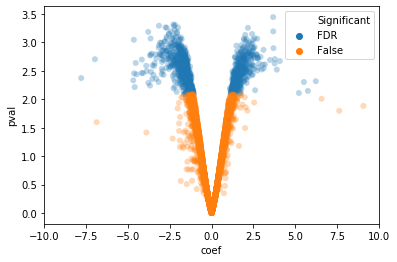#### 4.2.2 Biological interpretation¶

Similar to the biological interpretation of linear regression results, Alzheimer's hallmark proteins Amyloid beta and Tau protein are highly signficant. However, SMOC1 is not significant this time, despite its huge outlier beta-coefficient of 75 (in fact, it's the only outlier not shown in this volcano plot). Closer examination reveals that this is because of perfect separability, as demonstrated in the plot below. In perfect separability cases, logistic regression fails to give meaningful p-values.

In :
ADuniv_log.loc[("Q9H4F8-2", "P05067", "P10636", "P37840"),:]

Out:
Gene coef pval Significant
Accession
Q9H4F8-2 SMOC1 75.437876 0.677965 False
P05067 APP 3.675193 0.000359 FDR
P10636 MAPT 5.751427 0.007119 FDR
P37840 SNCA -0.433795 0.199033 False
In :
sns.stripplot(AD, proteome["Q9H4F8-2"], hue=AD)

Out:
<matplotlib.axes._subplots.AxesSubplot at 0x7f36a8f55438>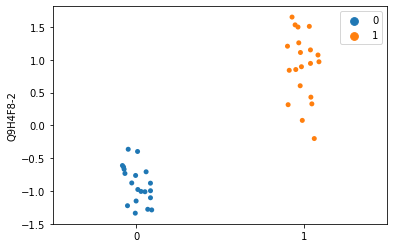### 4.3 Comparison of univariate linear- and logistic-regression results¶

It already becomes obvious by comparing the numbers of significant variables that linear-regression seems to be more powerful on this dataset: 132 Bonferroni- and 1623 FDR-significant proteins for linear-regression vs 0 Bonferroni- and 1016 FDR-significant proteins for logistic-regression (at the 0.05 level).

A more detailed interactive comparison of the two results are in the accompanying files 2_compare_univariate_results.ipynb and 2_compare_univariate_results.html.

## 5 Multivariate LASSO logistic regression¶

Remove SMOC1 (Q9H4F8-2) because of perfect separability. LASSO selects a subset of variables as predictors by penalizing on the L1-Norm of the beta-coefficients. SKLearn's LogisticRegressionCV employs cross-validation to identify the optimal lambda (or C) hyperparameter. Below are the selected proteins and their beta coefficients. The training-set size is 30 samples and the test set size is 10 samples.

In :
proteome_reduced = proteome.drop("Q9H4F8-2", axis=1)

X_train, X_test, y_train, y_test = train_test_split(proteome_reduced, AD, test_size=0.25, random_state=1337)

model = LogisticRegressionCV(cv=10, solver="liblinear", penalty="l1", random_state=1337).fit(X_train, y_train)

list(zip(proteome_reduced.columns[(model.coef_!=0)],
model.coef_[model.coef_!=0]))

Out:
[('Q15019-2', 0.07475893865168265),
('O75078', -0.050325227951352365),
('P05067', 0.17694675093618312),
('V9GYM8', -0.07648340129495527),
('P00966', 0.191924439342754),
('Q9UL15-2', -0.510364224138429),
('Q13490', -0.5421988317437187),
('Q9P1Z2', -0.03638737562594481),
('Q6P1J9', 0.004524336572358402),
('P02741', 0.09237296335569202),
('H7BYT1', 0.06420940778665873),
('Q96CG8', 0.39981851677520247),
('Q9UDY4', -0.11248206786135964),
('P07099', 0.0007605163156639481),
('P13726', 0.04195137694704598),
('Q86UX7', 0.07357031570028937),
('P60520', -0.0644233709563225),
('A6NHX0', -0.3453335016480302),
('Q9NZ52', -0.00860676289560626),
('Q8NDH6', -0.23816834785355184),
('Q14571', 0.0382484527026758),
('Q5SVJ7', -0.0004414865014175081),
('P18428', 0.006796543792457058),
('Q96NI6', -0.30309139344409874),
('Q5VZK9', 0.22035565656560557),
('Q8NAA5', -0.10402188465951608),
('P10636-6', 0.013450968474848817),
('E9PLM6', 0.26298961658960146),
('Q8WUY8', -0.006599971944642447),
('Q9Y697', -0.0006719433704057629),
('P01303', 0.23428022737294701),
('P01111', -0.1864771747887415),
('Q96F24', -0.008795708075730577),
('A0A087WWT2', -0.037268070955115605),
('Q15120-2', -0.0027301018340764385),
('Q96CD2', 0.21705705577837567),
('O75335', -0.03866794657308174),
('Q15435', -0.1671110534941245),
('P10586', -0.024519264845207858),
('Q15223', -0.04129885700265995),
('O75116', -0.3738244403852573),
('Q99250', -0.022262810404079087),
('P31947', 0.002606843462763877),
('P60880', -0.07169426434161981),
('Q15526', -0.04561358214852488),
('Q96B77', -0.12307483290713232)]

### 5.1 Test-set performance¶

In :
# train accuracy + confusion matrix
y_pred_class = model.predict(X_train)
print("Training accuracy:", metrics.accuracy_score(y_train, y_pred_class))
print("Training F1 score:", metrics.f1_score(y_train, y_pred_class))
#print("Log loss:", metrics.log_loss(y_train, y_pred_class))
#print(metrics.confusion_matrix(y_train, y_pred_class).transpose())

# test accuracy + confusion matrix
y_pred_class = model.predict(X_test)
print("Test-set accuracy:", metrics.accuracy_score(y_test, y_pred_class))
print("Test-set F1 score:", metrics.f1_score(y_test, y_pred_class))
#print("Log loss:", metrics.log_loss(y_test, y_pred_class))
#print(metrics.confusion_matrix(y_test, y_pred_class).transpose())

Training accuracy: 1.0
Training F1 score: 1.0
Test-set accuracy: 0.9
Test-set F1 score: 0.8571428571428571

In [ ]: